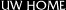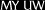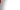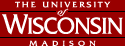Example of EES' CapabilitiesThe Problem: Consider refrigerant R-12 undergoing a throttling process. a) Given an inlet refrigerant temperature, pressure and velocity of 50 C, 700 kPa and 15 m/s, determine the outlet refrigerant temperature and velocity for a given outlet pressure of 300 kPa. b) Investigate the effect of varying the outlet pressure on the outlet temperature and velocity and plot the results. A Drawing package created the following figure to graphically describe the problem statement. EES allows the you to "Cut and Paste" into a diagram window.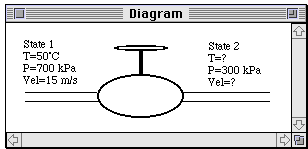The first thing you have to do is type in the equations into the Equations window. Typing equations is just like typing into a word processor. The equations can go in in any order and EES will automatically find the best way to solve the equations. Thermodynamic and transport property data for many fluids, such as R-12 used here, are provided with built-in functions. If you wish to see the equations formatted in a mathematical manner look at the Formatted Equations window. These equations can be cut and pasted into your favorite word processor. The Solution window contains the results for the outlet pressure equal to 300kPa. In order to see how the outlet pressure changes the outlet velocity and outlet temperature, a Parametric Table has been created. You put the bold numbers into the table and use the solve table command to find the outlet temperature and velocity for each outlet pressure. The results have been plotted in the Plot window.